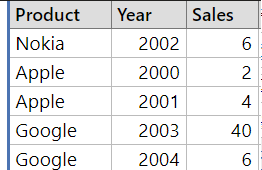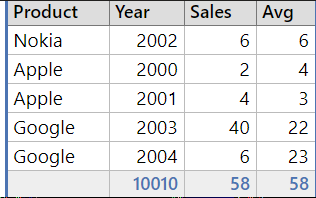# Moving Average In C# Using LINQ

This article will show you how to generate a moving average of C# collection using LINQ to Objects.

LINQ is a very popular feature of C# that allows the developer to write the query like SQL.

Let’s consider you have the following collection in C#. You want to calculate the moving average of the Sales column with the given window size.

InputFor example, Below Image shows the moving average of the Sales column with a window’s size of 2.

``````	Prdoduct=Apple,Year=2000
Avg=(6+2)/2=4
Product=Apple,Year=2001
Avg=(2+4)/2=3

``````

You got the idea ;) \$ads={1}

OutputLet’s create one extension method that calculates the moving average of numbers.

``````public static class MyExtensions
{

public static IEnumerable<double> MovingAverage(this IEnumerable<double> source, int windowSize)
{
var queue = new Queue<double>(windowSize);

foreach (double d in source)
{
if (queue.Count == windowSize)
{
queue.Dequeue();
}
queue.Enqueue(d);
yield return queue.Average();
}
}

}
``````
• The queue is initially empty.
• The queue is updated every time a new value is read from the source sequence.
• The average of all of the queued items is computed and returned.
• An item is removed from the queue once the appropriate sample size has been attained before a new one is added.
• This allows the sample to expand during the first few items but remains consistent once the optimal sample size is found.

Then we will use the `Zip` operator to calculate the average.  `Zip` Takes two collections and merges their corresponding values.

``````var results = sales
.Zip(sales.Select(s => s.Sales).MovingAverage(2),
(sale, value) => { sale.Avg = value; return sale; });
Console.WriteLine(results);
``````

Complete Source Code

``````void Main()
{
var sales = new List<SalesData>(){

new SalesData{Product="Nokia", Year=2002,Sales=6},
new SalesData{Product="Apple", Year=2000,Sales=2},
new SalesData{Product="Apple", Year=2001,Sales=4},

};

var results = sales
.Zip(sales.Select(s => s.Sales).MovingAverage(2),
(sale, value) => { sale.Avg = value; return sale; });
Console.WriteLine(results);
}

public class SalesData
{
public string Product { get; set; }
public int Year { get; set; }
public double Sales { get; set; }
public double Avg { get; set; }

}

``````